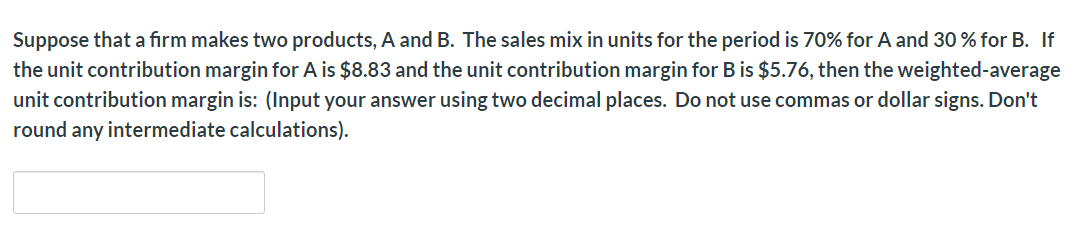# Suppose that a firm makes two products, A and B. The sales mix in units for the period is 70% for A and 30% for B. Ifthe unit contribution margin for A is \$8.83 and the unit contribution margin for B is \$5.76, then the weighted-averageunit contribution margin is: (Input your answer using two decimal places. Do not use commas or dollar signs. Don'tround any intermediate calculations)

Question
2 viewshelp_outlineImage TranscriptioncloseSuppose that a firm makes two products, A and B. The sales mix in units for the period is 70% for A and 30% for B. If the unit contribution margin for A is \$8.83 and the unit contribution margin for B is \$5.76, then the weighted-average unit contribution margin is: (Input your answer using two decimal places. Do not use commas or dollar signs. Don't round any intermediate calculations) fullscreen
check_circle

Step 1

Weighted average contribution margin:

The weighted average contribution margin is the average contribution margin of all the products contributed in a business...

### Want to see the full answer?

See Solution

#### Want to see this answer and more?

Solutions are written by subject experts who are available 24/7. Questions are typically answered within 1 hour.*

See Solution
*Response times may vary by subject and question.
Tagged in